Posts tagged ‘Bonn’

## L. Manivel: “Quantum Satake for Miniscule Spaces”

Here are my notes from Manivel’s talk in Bonn:

Manivel_Bonn_Sep_2010

Summary:

• geometric Satake after Drinfeld and Ginzburg
• example: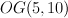$OG(5,10)$
• the quantum Chevalley formula (Fulton–Woodward)
• cohomology of G/P in general
• cohomology of G/P: improvements in the miniscule case
• semisimplicity of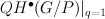$QH^\bullet(G/P)|_{q=1}$ (Chaput–Manivel–Perrin) and connections to work of Kostant (Gorbunov–Petrov)
• quantum Satake for miniscule type-D Grassmannians

## V. Golyshev: “Introduction to the Satake Correspondence”

Here are my notes from Golyshev’s second talk in Bonn:

Golyshev_2_Bonn_Sep_2010

Summary:

• the “spin representation of K3” and its quantum analog
• some evidence: the Bertram–Ciocan-Fontanine–Kim result for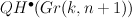$QH^\bullet(Gr(k,n+1))$
• the geometric Satake correspondence (Drinfeld–Ginzburg)
• miniscule Grassmannians as smooth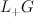$L_+ G$-orbits in the affine Grassmannian
• a quantum analog of geometric Satake in this case

## A. Kresch: “Quantum Cohomology of Miniscule Type-D Grassmannians”

Here are my notes from Kresch’s talk in Bonn:

Kresch_Bonn_Sep_2010

Summary:

• the orthogonal Grassmannian
• Schubert varieties are indexed by strict partitions
• special Schubert classes
• ring presentations for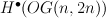$H^\bullet(OG(n,2n))$ and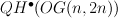$QH^\bullet(OG(n,2n))$
• the connection to algebraic combinatorics via Praguez-Rataiski polynomials
• quantum Giambelli and quantum Pieri (Kresch-Tamvarkis)

## L. Katzarkov: “Gaps, Spectra, and Applications”

Here are my notes from Katzarkov’s talk in Bonn:

Katzarkov_Bonn_Sep_2010

Summary:

• Clemens-Griffiths showed that the 3-dimensional cubic is not rational by showing that its intermediate Jacobian is not the Jacobian of a curve; we suggest analogs of this.
• Homological Mirror Symmetry and the perverse sheaf of vanishing cycles
• detecting rationality via monodromy properties on the LG mirror (Gross-Katzarkov, Pryzalkowski, Golyshev)
• spectra of triangulated categories; examples
• Theorem: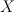$X$ is rational of dimension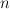$n$ implies that the spectrum of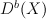$D^b(X)$ has no gaps of size greaeter than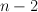$n-2$
• the outlook for 4-dimensional cubics

## V. Golyshev: “Quantum Motives: Linearizations, Realizations, Use, Detections”

Here are my notes from Golyshev’s talk in Bonn.

Golyshev_1_Bonn_Sep_2010

Summary:

• a historical analogy: the study of Fano varieties now versus algebraic number theory prior to the proof of the Weil Conjectures
• linearization and motives
• Tannakian categories
• the “quantum Tannakian category”
• realizations in algebraic number theory: one can detect algebraic varieties by detecting their motives (in practice by detecting their L-functions via Selberg-Stark)
• the “quantum Tate realization” and quantum detection
• the Satake correspondence and its quantum parallel